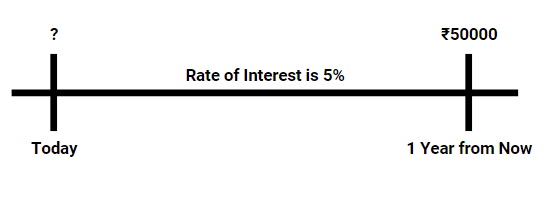# Present Value of a Single Cash Flow

The present value of single cash flow is used to determine the lumpsum investment requires to be done today at a certain rate of interest to meet certain expenses in a future date. E.g. investing in bank FD today to meet college fee liability after 2 years.
• In other words, Present Value is the Value in the Present, of a sum of money that is due to be received in the Future.

#### Consider the following Problem Statements

Example 1: Deepak has recently joined an IT Company, he plans to buy a bike after 1 year and so he
wants to invest some money today, he is not aware of the amount he needs to invest and so he goes to Bank and gets the information that Interest rate for 1-year Deposits is 5%
• The cost of the bike is Rs 50000, and the bank offers 5% Interest on Deposits for 1 year
• The above problem can be thought of as what amount invested today will become Rs 50000 after 1 year.• To calculate the above, there is a very simple formula:
PV = FV (1 + R) -T
• Where PV is the money to be invested today
• FV is the money expected at the end of a period (50000 in this case)
• R is the rate offered (5% in this case)
• T is the time for which amount is invested (1 Year)
• Hence, Deepak needs to invest = 50000/ (1+0.05) = 50000/1.05 = Rs 47619.05

• The above example can be written as the Present Value of to ₹50000.00 receivable after 1 year is Rs 47619.05 when the rate of interest is 5%.
• Now, by using the above formula we can easily find out the initial investment required to meet a final goal.
• The problem faced by Deepak is very common, everyone faces this while planning a future goal. While planning for a tour/education/marriage in the future we all know the possible expense but are keen to know the amount we should save today to meet that goal. The above formula will help in it.

#### Examples:

Example 2: Ramesh offers Ram to either accept Rs 5000 today or Rs 6000 after two years when the rate of interest is 5%. Ram is confused about which of them is the better scheme, let us help Ram.
• Solution: Present Value of Rs 5000 receivable today is Rs 5000
• But what is the present value of Rs 6000 receivables after 2 years? Let’s Calculate
• PV = 6000 (1 + 0.5) -2  = Rs 5442.18
• We can clearly see that the option to receive Rs 6000 after two years is more profitable than the first one as its Present value today is greater.

Example 3: Calculate the rate at which Rs20000 invested today will yield a maturity of Rs 24200 in 2 years.
• Solution: As given, PV = Rs 20000, FV= Rs 24200, T=2 years, R=?
• We know that, FV = PV (1 + R) T
• Substituting the values, 24200 = 20000(1 + R) 2  = 24200/20000 =(1 + R) 2
• This becomes, 1.21 =(1 + R) 2 , as we know 121 is square of 11, so 1+R = 1.1
• Hence Rate = 0.1 or 10%

### Graphical Representation of Present Values

• The following graph shows the variation in the present value of Rs 10000 receivable at various times in the future (3,5,7,8,9,10 years). For example, the present value of Rs 10000 receivable after 3 years is Rs 58638.38.